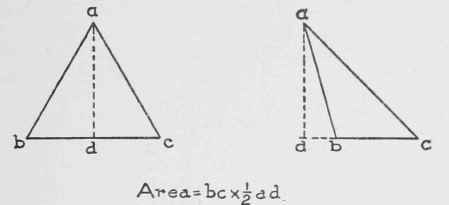1. To find the area of a parallelogram: Rule - Multiply the length by the breadth or perpendicular height. See Fig. 1Fig. 1.

2. To find the area of a triangle: Rule - Multiply the base by half the altitude. See Fig. 2.

3. To find the hypothenuse of a right-angled triangle when the base and perpendicular are known: Rule - Add together the square of the known sides and extract the square root of the sum. See Fig. 3.

4. To find one side of a right-angled triangle when the hypothenuse and the other side are known: Rule - From the square of the hypothenuse subtract the square of the given side, and the square root of the remainder will be the other side. See Fig. 4.Fig. 2.Fig. 3.Fig. 4.Fig. 5.

5. To find the area of a trapezium: Rule - Divide the figure into triangles by drawing a diagonal and the sum of the areas of these triangles will be the area of the trapezium. See Fig. 5.

6. To find the area of a trapezoid: Rule - Add the two parallel sides and mutliply by one-half the perpendicular distance between them. See Fig. 6.Fig. 6.Fig. 7.

7. To find the area of a regular polygon: Rule - Multiply one side by half its perpendicular distance from the center, and this product by the number of sides.

 Table of Multiples to Compute Measurements of Regular Polygons, theSide of the Polygon Being Unity Name of Polygon No. OFSides A Area BRadius of Circumscribing Circle CLength of theSide. D radius ofInscribedCircle Triangle... 3 0.433013 0.5773 1.732 0.2887 Tetragon.. 4 1 0.7071 1.4142 0.5 Pentagon.. 5 1.720477 0.8506 1.1756 0.6882 Hexagon.. 6 2.598076 1 1 0.866 Heptagon.. 7 3.633912 1.1524 0.8677 1.0383 Octagon___ 8 4.828427 1.3066 0.7653 1.2071 Nonagon.. 9 6.181824 1.4619 0.684 1.3737 Decagon. 10 7.694209 1.618 0.618 1.53S3 Undecagon.. 11 9.36564 1.7747 0.5634 1.7028 Dodecagon . 12 11.196152 1.9319 0.5176 1.S66

8. To find the area of a regular polygon when the length of a side only is given: Rule - Multiply the square of the side by the number opposite the name of the polygon in Column A.

9. To find the radius of a circumscribing circle when the length of a side only is given: Rule - Multiply the length of a side of the polygon by the number in Column B.

10. To find the length of side of a polygon that is contained in a given circle, when the radius of the circle is known: Rule - Multiply the radius of the circle by the number opposite the name of the polygon in Column C.

11. To find the radius of a circle that can be inscribed in a given polygon, when the length of a side is given: Rule - Multiply the length of a side of the polygon by the number opposite the name of the polygon in Column D.Fig. 8.Fig. 9.

12. To find the area of an irregular polygon: Rule - Divide the polygon into triangles and add the areas of all the triangles. Fig. 7.

13. To find the area of a ring included between the circumferences of two concentric circles: Rule - Square the diameters and multiply difference between the squares by .7854. Fig. 8.

14. To find the area of an ellipse: Rule-Multiply the two axes together and the product multiplied by .7854 will be the area.

15. To find the circumference of an ellipse: Rule - SquareFig. 10. Frustrum of Pyramid.